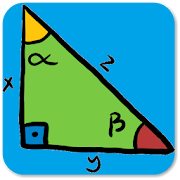# Math FormulasEveryone
This is a perfect app on Google Play that provides all basic formulas in mathematics. It's very convenient for all students in high school or university and engineers to look for any easy or complicated formulas. It includes:

All essentials math formula and equations:
Algebra
Geometry
Trigonometry

Calculus:
Limits
Derivatives
Integrals

Basic Properties & Facts

Arithmetic Operations
Exponent Properties
Properties of Inequalities
Properties of Absolute Value
Distance Formula
Complex Numbers
Logarithms and Log Properties
Factoring and Solving

Factoring Formulas
Square Root Property
Absolute Value Equations/Inequalities
Completing the Square
Functions and Graphs

Constant Function
Line/Linear Function
Circle
Ellipse
Hyperbola

Square
Rectangle
Circle
Triangles
Parallelogram
Trapezoid
Cube
Cylinder
Sphere
Cone
All in one
Geometric Symbols

Trigonometry Functions
Special Angles
Trigonometric Function Values in Quadrants II, III, and IV
The Unit Circle
Double Angle Formulas
Half Angle Formulas
Power Reducing Formulas
Product‐to‐Sum Formulas
Co-functions Formulas
Law of Sines
Law of Cosines
Law of Tangents
Pythagorean Identities (for any angle θ)
Mollweide’s Formula

Limits Definitions
Relationship between the limit and one-sided limits
Limits Properties Formulas
Basic Limit Evaluations Formulas
Evaluation Techniques Formulas
Some Continuous Functions
Intermediate Value Theorem

Derivatives Definition and Notation
Interpretation of the Derivative
Basic Properties and Formulas
Common Derivatives
Chain Rule Variants
Higher Order Derivatives
Implicit Differentiation
Increasing/Decreasing – Concave Up/Concave Down
Extrema
Mean Value Theorem
Newton’s Method
Related Rates
Optimization

Integrals Definitions
Fundamental Theorem of Calculus
Properties
Common Integrals
Standard Integration Techniques
Improper Integral
Approximating Definite Integrals

Math Formulas - a must-have app for your smartphones and tablets.

ef57a81c25
Collapse

## Reviews

Review Policy

Updated
August 3, 2017
Size
8.8M
Installs
1,000+
Current Version
1.0.1
Requires Android
4.0 and up
Content Rating
Everyone
Permissions
Offered By
Svey Development
Developer
Svey Development My Mail Box 889694 Singapore 919191